# CLstunfti¶

## Purpose of Module¶

CLstunfti is an extendable Python toolbox to compute scattering of electrons with a given kinetic energy in liquids and amorphous solids. It uses a continuum trajectory model with differential ionization and scattering cross sections as input to simulate the motion of the electrons through the medium.

Originally, CLstunfti was developed to simulate two experiments: A measurement of the effective attenuation length (EAL) of photoelectrons in liquid water  and a measurement of the photoelectron angular distribution (PAD) of photoelectrons in liquid water . These simulations were performed to determine the elastic mean free path (EMFP) and the inelastic mean free path (IMFP) of liquid water . Additionally, a program based on CLstunfti is currently being developed which simulates electron scattering in liquids in the presence of laser fields. This extension of CLstunfti is used for simulation of attosecond experiments in liquid water.

The EMFP and IMFP are two central theoretical parameters of every simulation of electron scattering in liquids, but they are not directly accessible experimentally. As CLstunfti can be used to determine the EMFP and IMFP from experimental data, and as it can be easily extended to simulate other problems of particle scattering in liquids, it was decided to make the source code publicly available. For thus purpose, within the E-CAM module a documentation for the code was written and examples were designed to test the code and learn how to use CLstunfti.

  Suzuki, Nishizawa, Kurahashi, Suzuki, Effective attenuation length of an electron in liquid water between 10 and 600 eV, Phys. Rev. E 90, 010302 (2014).
  Thürmer, Seidel, Faubel, Eberhardt, Hemminger, Bradforth, Winter, Photoelectron Angular Distributions from Liquid Water: Effects of Electron Scattering, Phys. Rev. Lett. 111, 173005 (2013).
  Schild, Peper, Perry, Rattenbacher, Wörner, An alternative approach for the determination of mean free paths of electron scattering in liquid water based on experimental data, submitted.

## Background Information¶

Within this E-CAM module, the necessary steps were taken to make CLstunfti a useful toolbox for other researchers by providing a documentation, examples, and also extensive inline documentation of the source code. CLstunfti is available at https://gitlab.com/axelschild/CLstunfti and is published together with the E-CAM module.

## Building and Testing¶

To use CLstunfti, the following steps are necessary:

• A few Python packages are needed. Specifically, you need:
• h5py==2.10.0
• matplotlib==3.2.2
• scipy==1.5.0
• numexpr==2.7.1
• numpy==1.19.0
• Move the main folder CLstunfti in a folder named e.g. My_Python_Modules. Then, either run

python setup.py build_ext --inplace


in the main folder or change to the CLstunfti subfolder and run

f2py -c --opt='-O3 -ffast-math' ftools.f95 -m ftools


to compile the Fortran code as a module. To make Python know where CLstunfti is, run

export PYTHONPATH=$PYTHONPATH:$HOME/My_Python_Modules/CLstunfti


in your shell or add the line to the end of your .bashrc (or .zshrc or .cshrc) file.

• Build the documentation by running

make html


It is found in _build/html (actually, it should already be there).

• Use CLstunfti!

The examples created for this E-CAM module are in the folder examples. Each example comes with a sample output which has the same name as the files created by the scripts, but appended with _ref. Some of the examples have a rather long runtime, as indicated below. This is because the examples should also show what is needed to compute the relevant targets correctly. If a quick test is preferred, the number of trajectories can be decreased.

The following examples are provided (note that part of the code is in the file tools_eal_pad.py in the example folder):

• Example 01 shows how to prepare an input for CLstunfti. It is run as

python 01_create_input.py


It will create the HDF5 file prop_data.h5 which can be compared with the reference file prop_data_ref.h5.

• Example 02 shows how to compute the effective attenuation length (EAL), i.e., the effective/average depth from which photoelectrons are ionized. This is done by selecting many ionization depths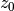and by fitting the number of electrons detected outside the liquid toIt is run as

python 02_compute_eal.py


and creates 02_eal.pdf which can be compared with 02_eal_ref.pdf. The calculation takes ca. 1 minute on a 3.40GHz CPU.

• Example 03 shows how to compute the photoelectron angular distribution (PAD) of electrons that leave the liquid after photoionization. This is done by rotating the PAD for photoionization (which simulates a rotation of the laser used for ionization) away from its default direction (the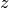-axis, as the default is that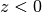is the liquid and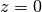is the surface) and by detecting the number of electrons outside the liquid depending on the polar angle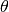of the rotation. The PAD has the functional form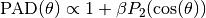where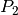is the Legendre polynomial of second order. Hence, the PAD is fully characterized by the parameter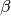.

Two ways to do the calculation are provided. The first uses importance sampling of the ionization depth with an exponential distribution, is run with

python 03a_compute_pad.py


and creates 03a_pad.pdf which can be compared with 03a_pad_ref.pdf. The calculation takes ca. 1 hour on a 3.40GHz CPU.

The second way uses a linear sampling, where initial positions are added until deeper and deeper in the liquid until no trajectories are leaving it anymore. It is run with

python 03b_compute_pad.py


and creates 03b_pad.pdf which can be compared with 03b_pad_ref.pdf. The calculation takes a few hours on a 3.40GHz CPU.

• Example 04 shows how to find elastic and inelastic mean free paths if an EAL and PAD are given. From an initial guess for the EMFP and IMFP, it optimizes their values by comparing the calculated EAL and PAD with a target EAL and PAD. It is run with

python 04_find_emfp_imfp.py


and provides the terminal output given in 04_find_emfp_imfp_output.txt for comparison. The calculation takes ca. 1 hour on a 3.40GHz CPU.

• Example 05 compares the angular distribution of photoelectrons after ionization, one scattering, two scatterings, etc. in the bulk (no surface) with the known solution. There are four parts. The calculations should be performed in the right order because the results are saved to files.

In the first part, the angular distribution of the electrons after up to nine scatterings in the bulk without inelastic scattering is computed. It is run with

python 05a_bulk_prep.py


and creates 05a_bulk.pdf and 05a_bulk.h5 which can be compared with 05a_bulk_ref.pdf and 05a_bulk_ref.h5, respectively. The calculation takes ca. 1.5 hours on a 3.40GHz CPU.

In the second part, results of the first part are compared with a convolution of the initial PAD with the DSCS and with doing the exact equivalent of the convolution (the convolution only gives the exact result in 2D, in 3D it is more complicated). It is run with

python 05b_compare_bulk_convolution.py


and creates 05b_compare_bulk_convolution.pdf which can be compared with 05b_compare_bulk_convolution_ref.pdf. The calculation takes a few seconds on a 3.40GHz CPU.

In the third part, the angular distribution of the electrons after up to nine scatterings is computed outside the surface. It is run with

python 05c_surface.py


and creates 05c_surface.pdf which can be compared with 05c_surface_ref.pdf. The calculation takes ca. 10 minutes on a 3.40GHz CPU.

In the fourth part, the results of the first and third part are compared. It is run with

python 05d_comparison_bulk_surface.py


and creates 05d_comparison_bulk_surface.pdf which can be compared with 05d_comparison_bulk_surface_ref.pdf. The calculation takes a few seconds on a 3.40GHz CPU.

## Source Code¶

For the module, the documentation and the examples were developed and the source code of CLstunfti was extensively commented.